4.12.1 From Tree to Vine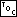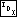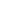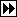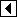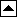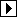The first stage of balancing converts a binary tree into a linear structure resembling a linked list, called a vine (see vine). The vines we will create have the greatest value in the binary tree at the root and decrease descending to the left. Any binary search tree that contains a particular set of values, no matter its shape, corresponds to the same vine of this type. For instance, all binary search trees of the integers 0...4 will be transformed into the following vine: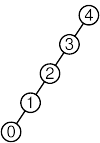The method for transforming a tree into a vine of this type is similar to that used for destroying a tree by rotation (see Destroying a BST by Rotation). We step pointer p through the tree, starting at the root of the tree, maintaining pointer q as p's parent. (Because we're building a vine, p is always the left child of q.) At each step, we do one of two things:

• If p has no right child, then this part of the tree is already the shape we want it to be. We step p and q down to the left and continue.
• If p has a right child r, then we rotate left at p, performing the following transformation:

where a, b, and c are arbitrary subtrees or empty trees. Node r then becomes the new p. If c is an empty tree, then, in the next step, we will continue down the tree. Otherwise, the right subtree of p is smaller (contains fewer nodes) than previously, so we're on the right track.

This is all it takes:

```89. <BST to vine function 89> =
/* Converts tree into a vine. */
static void tree_to_vine (struct bst_table *tree) {
struct bst_node *q, *p;

q = (struct bst_node *) &tree->bst_root;
p = tree->bst_root;
while (p != NULL)
if (p->bst_link == NULL)       {
q = p;
}
else       {
struct bst_node *r = p->bst_link;